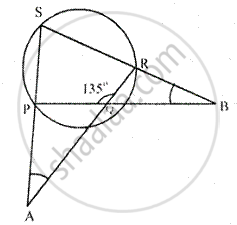Share

In a Cyclic – Quadrilateral Pqrs, Angle Pqr = 135°. Sides Sp and Rq Produced Meet at Point a : Whereas Sides Pq and Sr Produced Meet at Point B. If ∠A : ∠B = 2 : 1; Find Angles a and B. - ICSE Class 10 - Mathematics

Question

In a cyclic – quadrilateral PQRS, angle PQR = 135°. Sides SP and RQ produced meet at point A : whereas sides PQ and SR produced meet at point B.
If ∠A : ∠B = 2 : 1; find angles A and B.

SolutionPQRS is a cyclic quadrilateral in which ∠PQR = 135°
Sides SP and RQ are produced to meet at A and Sides PQ and SR are produced to meet at B.
∠A = ∠B = 2 : 1
Let ∠A = 2X , then ∠B - X
Now, in cyclic quad PQRS,
Since, ∠PQR = 135°,  = 180° -135°  = 45°
[since sum of opposite angles of a cyclic quadrilateral are supplementary]
Since, ∠PQR and ∠PQA are linear pair,
∠PQR + ∠PQA = 180°
⇒ 135° + PQA = 180°
⇒ ∠PQA = 180° -135° = 45°

Now, In ∆PBS,
∠P = 180° - (45° + x ) = 180° - 45° - x = 135° - x      …..(1)
Again, in ∆PQA,

EXT ∠P = ∠PQA + ∠ = 45° + 2X     ……….(2)
From (1) and (2),
45° + 2x =135° - x
⇒ 2x + x = 135° - 45°
⇒ 3x = 90°
⇒  x = 30°
Hence, ∠A = 2x = 2 ×30° = 60°
And ∠B = x = 30°

Is there an error in this question or solution?
Solution In a Cyclic – Quadrilateral Pqrs, Angle Pqr = 135°. Sides Sp and Rq Produced Meet at Point a : Whereas Sides Pq and Sr Produced Meet at Point B. If ∠A : ∠B = 2 : 1; Find Angles a and B. Concept: Cyclic Properties.
S